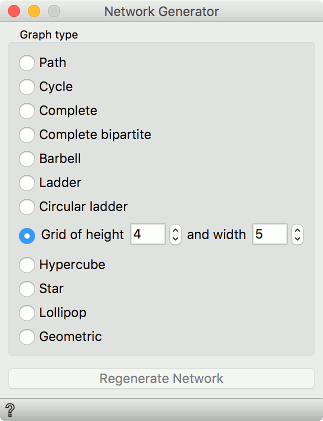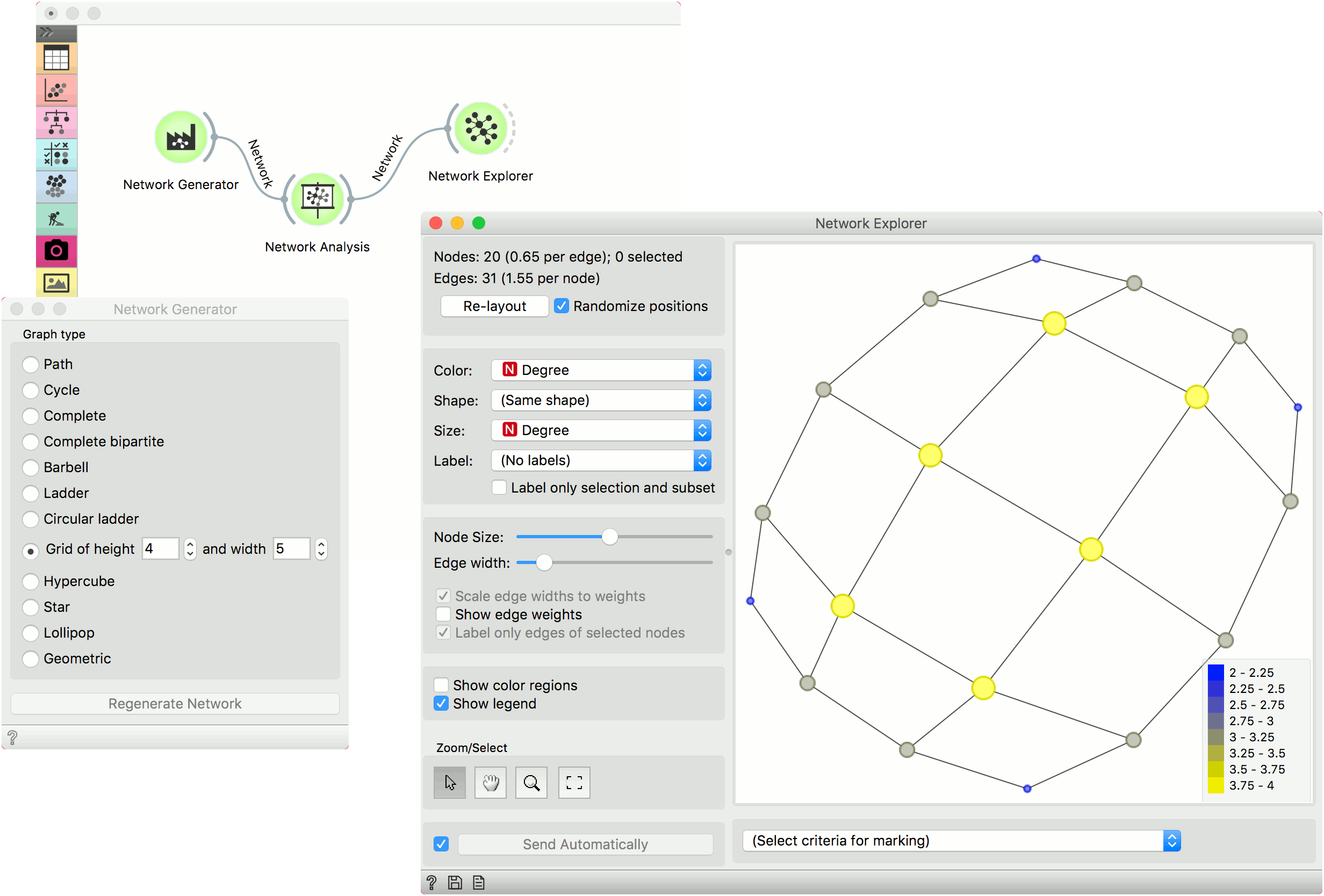# Network Generator

Construct example graphs.

Outputs

• Generated Network: An instance of Network Graph.

Network Generator constructs exemplary networks. It is mostly intended for teaching/learning about networks.Graph options:

• Path: a graph that can be drawn so that all of its vertices and edges lie on a single straight line.
• Cycle: a graph that consists of a single cycle, i.e. some number of vertices (at least 3) are connected in a closed chain.
• Complete: simple undirected graph in which every pair of distinct vertices is connected by a unique edge.
• Complete bipartite: a graph whose vertices can be divided into two disjoint and independent sets.
• Barbell: two complete graphs connected by a path.
• Ladder: planar undirected graph with 2n vertices and 3n-2 edges.
• Circular ladder: Cartesian product of two path graphs.
• Grid: a graph whose drawing, embedded in some Euclidean space, forms a regular tiling.
• Hypercube: a graph formed from the vertices and edges of an n-dimensional hypercube.
• Star: Return the Star graph with n+1 nodes: one center node, connected to n outer nodes.
• Lollipop: a complete graph (clique) and a path graph, connected with a bridge.
• Geometric: an undirected graph constructed by randomly placing N nodes in some metric space.

Press Regenerate Network to output a new graph instance.

## Example

Network Generator is a nice tool to explore typical graph structures.Here, we generated a Grid graph of height 4 and width 5 and sent it to Network Analysis. We computed node degrees and sent the data to Network Explorer. Finally, we observed the generated graph in the visualization and set the size and color of the nodes to Degree. This is a nice tool to observe and explain the properties of networks.